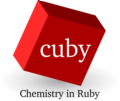back to Tutorials

# First calculation tutorial

Following series of calculation can be performed without any additional software, it uses an forcefield for water built in cuby (interface water_ff). We will use it to optimize water dimer and calculate interaction energy and vibrational frequencies on the resulting structure.

We will start with not very good geometry stored in file water_dimer.xyz

``````6

O   -1.289980673  -0.074565899   0.043046094
H   -1.840150791   0.820446452  -0.077860398
H   -0.357814959   0.190558911   0.040426166
O    1.608352477   0.145730472  -0.013823657
H    1.599659059  -0.364660368  -0.760371972
H    1.519660907  -0.497227172   0.798024829``````

The same input file can be used for all the calculations. The calculations are run using different modules. The calculation setup (selecting the interface and specifying charge of the system) is shared, but each module uses its specific keywords from the rest of the input and ignores the others.

Here is the input file (in YAML format), let's save it as input.yaml

``````# Interface
interface: water_ff
# Net charge
charge: 0

# Keywords for optimization
opt_quality: 0.1
restart_file: restart.xyz

# Keywords for frequencies calculation
numerical_hessian: yes
numerical_hessian_d: 0.0005
``````

## Geometry optimization

Running module cuby_optimize:

``cuby_optimize input.yaml water_dimer.xyz | tee LOG.optimize``

will perform the optimization. We have requested tight convergence criteria using the opt_quality keyword to get good starting point for the frequency calculation. We redirect output of the module to both screen and file LOG.optimize using the tee command. The log contains records on each step of the optimization and we will use it to plot the energy during the optimization. Cuby comes with a simple script that can extract the energies from the log file. Following command will plot them using xmgrace:

``plotlog LOG.optimize | xmgrace -``

The module cuby_optimize also produces a file history.xyz (this is the default filename, it can be changed by keyword history_file) containing geometry of the system in each step of optimization. Any visualization program can be used to view the file.

Finally, we requested in the input saving the final geometry to file restart.xyz. We will use this geometry in the following calculations

## Interaction energy

The module cuby_interaction calculates interaction energy between two molecules. To handle the most common calculations with only a simple input file, cuby uses following procedure: When no additional information is found in the input, it attempts to find separate molecules in the geometry, and if there are two, interaction between them is calculated. If the total charge of the dimer is zero and no information is provided on charge of the subsystems, they are assumed to be neutral too. Running

``cuby_interaction input.yaml restart.xyz``

will print the interaction energy.

In some cases, we don't need the fancy decorations of the output. For automated processing of the results, we'd prefer to get only the number. It can be achieved by changing the verbosity of the output. At verbosity level 1, only minimum information is printed out:

``cuby_interaction -v 1 input.yaml restart.xyz``

## Frequencies

The interface does not provide analytical calculation of second derivatives of the energy, so we have to use numerical differentiation, invoked by keyword numerical_hessian. The value of numerical_hessian_d specifies the displacement (in Angstroms) used in this calculation.

Module cuby_freq prints vibrational energies and calculated zero-point vibrational energy (ZPVE) to the output:

``cuby_freq input.yaml restart.xyz``

In addition, it writes file 'Molden.input' that contains the output in format that can be opened in molden for visualization of the normal modes.